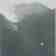Lava-Cooling Operations During the 1973 Eruption of Eldfell Volcano,
Heimaey, Vestmannaeyjar, Iceland
U.S. Geological Survey Open-File Report 97-724

 Table 1. Summary of pumps [deployed for lava cooling] Figure 7.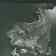Figure 16.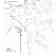Table 2. Types of jobs and number employed in lava-cooling operations between April and June 1973 Figure 8.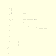Figure 17.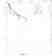Table 3. Summary of cost 6 of lava cooling [operations] Figure 9.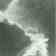Figure 18.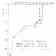Figure 1.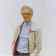Figure 10.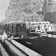Figure 19.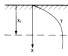Figure 2.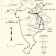Figure 11.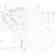Figure 20.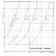Figure 3.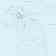Figure 12.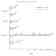Figure 21.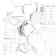Figure 4.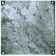Figure 13.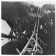Figure 22.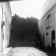Figure 5.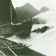Figure 14.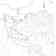Figure 23.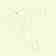Figure 6.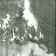Figure 15.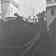Figure 24.